# Percentages - examples - page 5

1. Event probability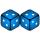The probability of event P in 8 independent experiments is 0.33. What is the probability that the event P occurs in one experiment (probability is same)?
2. Triangle KLBIt is given equilateral triangle ABC. From point L which is the midpoint of the side BC of the triangle it is drwn perpendicular to the side AB. Intersection of perpendicular and the side AB is point K. How many % of the area of the triangle ABC is area o
3. Machine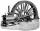Price of the new machine is € 62000. Every year is depreciated 15% of residual value. What will be the value of the machine after 3 years?
4. Glass mosaicHow many dm2 glass is nessesary to produc 97 slides of a regular 6-gon, whose side has length 21 cm? Assume that cutting glass waste is 10%.
5. Potatoes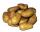Potatoes contain 78.6% starch. How many potatoes need to obtain 27 kg of starch?
6. Shooter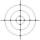The probability that a good shooter hits the center of the target circle I is 0.1. The probability that the target hit intercircle is II 0.58. What is the probability that it hits the target circle I or II?
7. Paddlers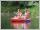Students of canoeists trip traveled in three days 102 km. Second day traveled 15% more than first day and at the third day 9 km over the second day. How many kilometers traveled each day?
8. Events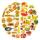Event N has probability of 0.24. What is the probability that the event N occurs in 8, 5, 4 try.
9. Sales vs profit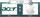Apple sells 22 percent less computes than the Acer but the profit from their sales is 2.2 times higher than Acer. How many times are Apple computer more expensive than Acer?
10. Water containerContainer with water weighs 1.48 kg. When we cast 75% of water container of water weight 0.73 kg. How heavy is an empty container?
11. Company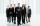After increasing the number of employees by 15% company has 253 employees. How many employees take?
12. Bureaucracy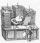The office was attended by 23 people. 9 of them completed the questionnaire. What is the percentage of all people?
13. Insurance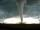The owner of the house is insured against natural disasters and pays 0.04% annually of the value of house 77 Eur. Calculate the value of the house. Calculate the probability of disaster, if you know that 48% of the insurance is to pay damages.
14. Cone in cylinder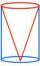The cylinder is inscribed cone. Determine the ratio of the volume of cone and cylinder. The ratio express as a decimal number and as percentage.
15. IcerinkRectangular rink with dimensions of 68.7 m and 561 dm must be covered with a layer of ice 4.2 cm thick. How many liters of water is necessary for the formation of ice when the volume of ice is 9.7% greater than the volume of water.
16. Apartments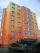Apartment on the first floor was 10% more expensive than the same apartment on the second floor. The difference was 105 Kc annually. Calculate the annual rent of the apartment in the first floor and from apartment on the second floor.
17. DepositIf you deposit 959 euros the beginning of each year, how much money we have at 2.1% (compound) interest after 19 years?
18. Acids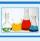70% acid was make from same acid of two different concentrations. Amount weaker acid to the stronger acid is in ratio 2:1. What was the concentration of the weaker acid, if stronger had 91% concentration?
19. VintnerHow high can vintner fill keg with crushed red grapes if these grapes occupy a volume of 20 percent? Keg is cylindrical with a diameter of the base 1 m and a volume 9.42 hl. Start from the premise that says that fermentation will fill the keg (the number.
20. Tesco Clubcard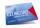Bernard got on a quarterly billing vouchers for Tesco ClubCard taking goods worth 2.3 Eur. Calculate how much per month on average in Tesco bought goods. Tesco ClubCard worksfor every Euro spent you get one ClubCard point that will be converted to 1 e

Do you have an interesting mathematical example that you can't solve it? Enter it, and we can try to solve it.

To this e-mail address, we will reply solution; solved examples are also published here. Please enter e-mail correctly and check whether you don't have a full mailbox.

Our percentage calculator will help you quickly calculate various typical tasks with percentages.